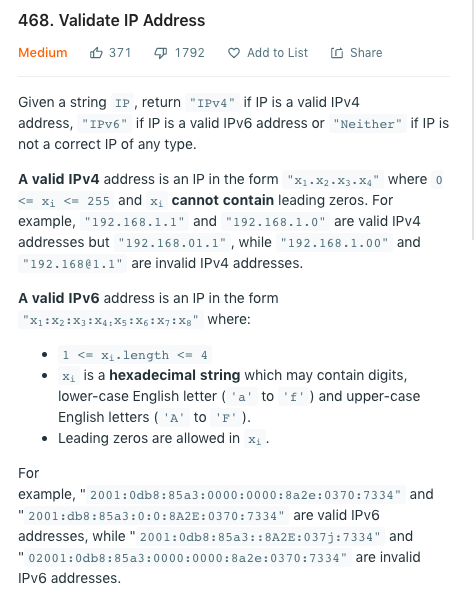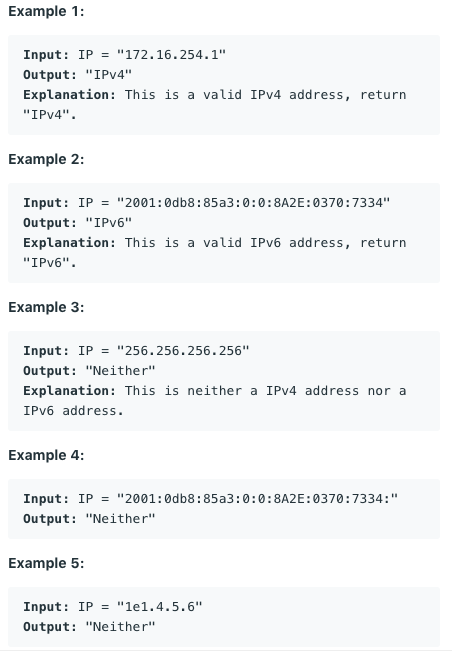## substring

subtring 跟以往 Java 使用的方式其實差不多

• 這裡首先用 indexOf 取得 `‘` 的 index
• until 這個 ranges function，讓我們寫起來更有語意上的感覺，也就是要取 index 0 ~ 3 的子字串，也就是 "Tim"
• 下面兩種寫法就跟 Java 內的方式一樣
``````val str = "Tim's string"
val index = str.indexOf('\'')

println(str.substring(0 until index)) // Tim
println(str.substring(0)) // Tim's string
println(str.substring(0, index)) // Tim
``````

## split

``````val names = "tim, jerry, anna"

val data = names.split(',') // ["tim", "jerry", "anna"]
val tim = data   // "tim"
val jerry = data // "jerry"
val anna = data  //"anna"
``````

## 解構 (destructuring)

``````val (tim, jerry, anna) = names.split(',')
``````

``````val (tim2, _, anna2) = names.split(',')
``````

## Nullable 的時候

Kotlin 很貼心，幾乎都內建好方法了，搭配之前提到的 `?.` 更方便，終於可以不用依靠一些 lib 了...

• isEmpty() 指得是真的長度為 0 的空字串
• 因為 isEmpty(), isNotEmpty() 並沒有判斷 null，所以需要 `? Safe Calls` 符號來判斷，如果是 null 就不會呼叫下去
• isNullOrEmpty() 就會包含 null 的判斷所以不需要 ? Safe Calls 符號來判斷 null
``````val s: String? = "kotlin"
// isEmpty, isNotEmpty 要有 ?, isNullOrEmpty 不需要
println(s?.isEmpty()) // length == 0
println(s?.isNotEmpty())
println(s.isNullOrEmpty())
``````

isBlank() 指得是有空白的字串或長度為 0 的空字串

``````// isBlank, isNotBlank 要有 ?, isNullOrBlank 不需要
println(s?.isBlank()) //  length == 0 或有空白
println(s?.isNotBlank())
println(s.isNullOrBlank())
``````

## 字串相接

``````println(s + " very good")
println(s.plus(" very good"))
println(s.plus(" very good").plus("haha"))
println("\$s very good")
``````

## StringBuilder

``````val builder = StringBuilder()
builder.append("Aloha")
.append(" ")
.append("Tim")
``````

## String template 會幫你優化成 StringBuilder

``````val a = "Aloha"
val b = " "
val c = "Tim"
val d = "\$a\$b\$c"
// 會幫我們轉成 (new StringBuilder()).append(a).append(b).append(c).toString();
``````

``````println("\$s very good")
// var19 = s + " very good"; 只有一次的字串串接, 還是會只用 +
``````

## Leetcode 344. Reverse String

https://leetcode.com/problems/reverse-string/

``````fun reverseString(s: CharArray): Unit {
var left = 0
var right = s.size - 1
while (left < right) {
val temp = s[left]
s[left] = s[right]
s[right] = temp
left++
right--
}
}
``````

``````fun reverseString(s: CharArray): Unit {
if (s.isEmpty()) return
for (i in 0..s.lastIndex/2) {
val temp = s[i]
s[i] = s[s.lastIndex - i]
s[s.lastIndex - i] = temp
}
}
``````

## Leetcode 468. Validate IP Address

https://leetcode.com/problems/validate-ip-address/• ipv4 由 4 組數字用 . 號隔開:
• 內容都是數字會介於 0~255，，所以要排除有文字的例外狀況
• ipv6 由 8 組文數字用 : 隔開:
• 內容是 16進位字串
• 所以內容會有
• 數字 和 字母 'a' 到 'f' 和大寫的 字母 'A' 到 'F' (因為 16進位是到 F )
• 可以 0 開頭
• 長度可以 1~4

``````fun validIPAddress(IP: String): String {
return try {
when {
IP.count { it == '.' } == 3 -> validateIPv4(IP)
IP.count { it == ':' } == 7 -> validateIPv6(IP)
else -> "Neither"
}
} catch (e: Exception) {
"Neither"
}
}
``````

`i !in 0..255` 檢查數字是否落在 0~255

`it != i.toString()` 把原本的 ip 和 ip 轉成數字再轉成字串做比較，看是不是轉換後還是一樣，用來處理一些特殊的 test case，像是 01.01.01.01

``````// it != i.toString() 處理一些特殊的 test case, 像是 01.01.01.01
private fun validateIPv4(IP: String): String {
IP.split('.').forEach {
val i = it.toInt()
if (i !in 0..255 || it != i.toString()) {
return "Neither"
}
}
return "IPv4"
}
``````

ipv6 的部分就判斷

``````private fun validateIPv6(IP: String): String {
IP.split(':').forEach {
if (it.length > 4 || !it.contains(Regex("^[0-9A-Fa-f]+\\$"))) {
return "Neither"
}
}
return "IPv6"
}
``````

``````class Solution {
fun validIPAddress(IP: String): String {
return try {
when {
IP.count { it == '.' } == 3 -> validateIPv4(IP)
IP.count { it == ':' } == 7 -> validateIPv6(IP)
else -> "Neither"
}
} catch (e: Exception) {
"Neither"
}
}

// it != i.toString() 處理一些特殊的 test case, 像是 01.01.01.01
private fun validateIPv4(IP: String): String {
IP.split('.').forEach {
val i = it.toInt()
if (i !in 0..255 || it != i.toString()) {
return "Neither"
}
}
return "IPv4"
}

private fun validateIPv6(IP: String): String {
IP.split(':').forEach {
if (it.length > 4 || !it.contains(Regex("^[0-9A-Fa-f]+\\$"))) {
return "Neither"
}
}
return "IPv6"
}
}
``````